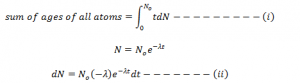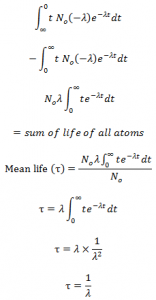# Mean Life Or Average Life

Radioactive atoms disintegrate spontaneously and it is not possible to predict which atom is going to disintegrate next. So, in order to deal this type of difficulty calculation mean life or average life of radioactive substance is introduced.
The atom which disintegrates at first is said to have zero (0) life and the atom which disintegrate last is said to have infinite life.
SO the life of radioactive atoms ranges from 0-infinity mean life gives the sum of the life of all the atoms to the total no. of atoms present initially.
Mathematically, it can be expressed as:
Mean life (τ) = sum of life of all atom / total no of atoms present
Mathematical calculation shows that mean life of radioactive substance is reciprocal of decay constant,
Mean life = 1/ decay constant
Derivation of mean life:
Let us consider, N0 be the total number of radioactive atoms present initially. After time t , total no. of atoms present (undecayed) be N . IN further dt time dN be the no. of atoms disintegrated. So, the life of dN atoms ranges lies between t + dt and dt. Since, dt is very small time, the most appropriate life of dN atom is t . So the total life of N atom = t. dNNow substituting the value of dN and changing the limit in equation i from ii, we get,This expression gives the relation between mean life and decay constant. Hence, mean life is reciprocal of decay constant.